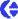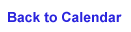### 9 March, 2002

Weekly Poker Flat Trip Martin, Lynette and I went to Poker Flat with three Fairbanks teachers to do the weekly measurements on four ponds: MST, 31.6, 33.5, and Jalpertia. As sunny as it usually

is, the wind traversed over Poker Flat at the speed of 25 mph bringing down the wind chill to ~5 F.

Let's Talk Science: Density Review On March 5th, I provided a brief explanation on snow density. To review, we need two

measurements to calculate density: snow samples and snow depth. The formula for density is mass per unit volume (D=M/V) and is expressed in g/cm3. Before we can proceed with the formula, we must find the volume of the snow sample. Volume is pi r2 h. The value for pi r2

is 21.711909 cm2, we multiply this value to the snow depth (h). Below are two measurements from today:

P1: 91.3 g / 21.711909 cm2 x 24.5 P4: 109.9 g / 21.711909 cm2 x 32 For answers, refer to March 10th journal entry.

Ice Thickness Ice thickness (Zi) is measured using either two methods: drilling or hot wire gauge. For a diagram and more information on hot wire gauges, refer to the January 24th journal entry.

Drilling Measurements are taken using a metal rod and meter stick.

Hot wire gauge A long resistance wire is attached to a metal toggle through the L-shaped, aluminum tube that is staked into the ice. L1 and L2 measurements are added and then subtracted from the total length.

What are the ice thickness calculations for the following measurements? Total Length = 245 cm

1. L1 = 104 cm, L2 = 53 cm 2. L1 = 134 cm, L2 = 48 cm 3. L1 = 123 cm, L2 = 39 cm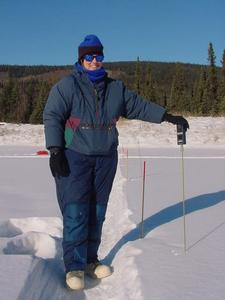1. Carol, middle school teacher takes base temperature.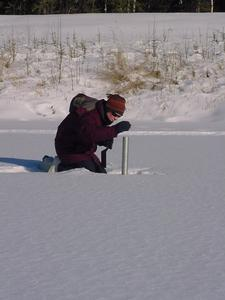2. Snow sample from P1.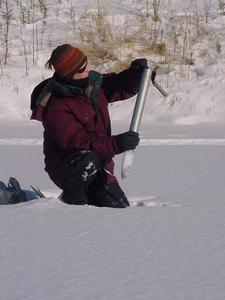3. Snow sample into plastic bag.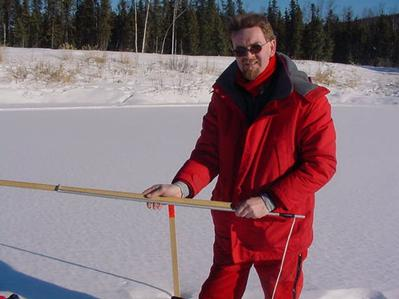4. Metal rod used to measure ice thickness (Zi) Ice Thickness Answers
1. 88 cm
2. 63 cm
3. 83 cmContact the TEA in the field at .
If you cannot connect through your browser, copy the TEA's e-mail address in the "To:" line of your favorite e-mail package.